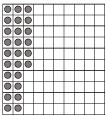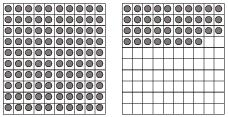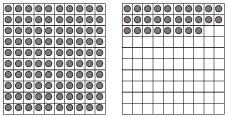# NCERT Solutions for Class 6 Maths Decimals Chapter 7 Exercise 8.2 and 8.3

In this page we have NCERT Solutions for Class 6 Maths Decimals Chapter 7 Exercise 8.2 and 8.3. Hope you like them and do not forget to like , social share and comment at the end of the page.

## Exercise   8.2

Question 1

Complete the table with the help of these boxes and use decimals to write the number.

(a)(b)(c)Ones Tenths Hundredths Number (a) - - - - (b) - - - - (c) - - - -

First counting the boxes in each figure we get

a) There are two tenths and 6 hundredths. So, the decimal is 0.26

b)      One whole or ones, 3 tenths and 8 hundredths. Decimal is 1.38

c)      One whole or ones, two tenths and 8 hundredths. Decimal is 1.28

 Ones Tenths Hundredths Number (a) 0 2 6 .26 (b) 1 3 8 1.38 (c) 1 2 8 1.28

Question 2

Write the numbers given in the following place value table in decimal form.
 Hundreds 100 Tens 10 Ones 1 Tenths Hundredths Thousandths (a) 0 0 3 2 5 0 (b) 1 0 2 6 3 0 (c) 0 3 0 0 2 5 (d) 2 1 1 9 0 2 (e) 0 1 2 2 4 1

 a) $0+0+3+\frac {2}{10}+\frac {5}{100}+ \frac {0}{1000}=3+ .2+ .05=3.25$ b) $100+0+2+ \frac {6}{10} + \frac {3}{100} + \frac {0}{1000}= 100+2+.6+.03= 102.63$ c) $0+30+0+0+ \frac {2}{100} + \frac {5}{1000}=30+ +.02+.005=30.025$ d) $200+ 10+1+ \frac {9}{10} + \frac {0}{100} + \frac {2}{10000}= 211+.9+.002=211.902$ e) $0+ 10+2+ \frac {2}{10} + \frac {4}{100} + \frac {1}{1000}= 12+.2+.04+.001= 12.241$

Question 3

Write the following decimals in the place value table.

(a) 0.29

(b) 2.08

(c) 19.60

(d) 148.32

(e) 200.812

 Hundreds 100 Tens 10 Ones 1 Tenths Hundredths Thousandths (a) 0 0 0 2 9 0 (b) 0 0 2 0 8 0 (c) 0 1 9 6 0 0 (d) 1 4 8 3 2 0 (e) 2 0 0 8 1 2

Question 4

Write each of the following decimals.

(a)$20 + 9 + \frac {4}{10} + \frac {1}{100}$

(b)$137 + \frac {5}{100}$

(c)$\frac {7}{10} + \frac {6}{100} + \frac {4}{1000}$

(d)$23 + \frac {2}{10} + \frac {6}{1000}$

(e)$700 + 20 + 5 + \frac {9}{100}$

 a) $20 + 9 + \frac {4}{10} + \frac {1}{100}$ 29+.4+.01=29.41 b) $137 + \frac {5}{100}$ 137+.05= 137.05 c) $\frac {7}{10} + \frac {6}{100} + \frac {4}{1000}$ .7+.06+.004=.764 d) $23 + \frac {2}{10} + \frac {6}{1000}$ 23+.2+.006=23.206 e) $700 + 20 + 5 + \frac {9}{100}$ 725+.09=725.09

Question 5

Write each of the following decimals in words.

(a) 0.03

(b) 1.20

(c) 108.56

(d) 10.07

(e) 0.032

(f) 5.008

a)      0.03 = zero point zero three

b)      1.20 = one point two zero

c)      108.56 = one hundred eight point five six.

d)     10.07 = ten point zero seven.

e)      0.032 = zero point zero three two

f)       5.008 = five point zero zero eight

Question 6

Between which two numbers in tenths place on the number line does each of the given number lie?

(a) 0.06

(b) 0.45

(c) 0.19

(d) 0.66

(e) 0.92

(f) 0.57

1.      0.06 lies between 0 and 0.1

2.      0.45 lies between 0.4 and 0.5

3.      0.19 lies between 0.1 and 0.2

4.      0.66 lies between 0.6 and 0.7

5.      0.92 lies between 0.9 and 1

6.      0.57 lies between 0.5 and 0.6

Question 7

Write as fractions in lowest terms.

(a) 0.60

(b) 0.05

(c) 0.75

(d) 0.18

(e) 0.25

(f) 0.125

(g) 0.066

a) .60= 60/100=3/5
b)  .05= 5/100=1/20
c) .75= 75/100= 3/4
d) .18= 18/100=9/50
e) .25= 25/100=1/4
f) .125= 125/1000=1/8
g) .066= 66/1000=      33/500

# Exercise 8.3

Question 1

Which is greater?

(a) 0.3 or 0.4

(b) 0.07 or 0.02

(c) 3 or 0.8

(d) 0.5 or 0.05

(e) 1.23 or 1.2

(f) 0.099 or 0.19

(g) 1.5 or 1.50

(h) 1.431 or 1.490

(i) 3.3 or 3.300

(j) 5.64 or 5.603

To find larger number, we can follow these steps

1) When comparing two positive decimals, here are the tips
 a) If two numbers have unequal number of digits on the whole part, then the number with the greater number of digits is greater. b) If two numbers have equal number of digits on the whole part then, the number with greater valued digit on the extreme left is greater. If the digits on extreme left of the numbers are equal, then the digits to the right of the extreme left digits are compared and so on. c)  if the whole part is same, then the number with a greater value digit at tenth part is greater, if the digits on tenth part are equal, then the digit on hundredth part compared and so on

2)  When comparing two negative decimals, we can remove the sign and compare them as positive integer given above, the smallest of them would be the greater when we put the sign back

3)We comparing two different sign decimals, the number with positive sign is larger

With that in mind, lets solve the above questions

a)      0.3 and 0.4

Apply the same principle as given above

The whole number part is the same. It can be seen that the tenth part (3) of 0.3 is less than the tenth part (4) of 0.4.

So, 0.4 is greater.

b)      0.07 and 0.02

Apply the same principle as given above

The whole number part is the same. It can be seen that the hundredth part (7) of 0.07 is greater than the hundredth part (2) of 0.02.

So, 0.07 is greater.

c)      3 or 0.8

Apply the same principle as given above

The whole part 3 is greater than the decimal 0.8

So, 3 is greater

d)     0.5 or 0.05

Apply the same principle as given above

The whole part is the same. Tenth part of 0.5 is 5 and the tenth part of 0.05 is 0. So, we see that the tenth part of 0.5 is greater than the tenth part of 0.05. So, 0.5 is greater.

e)      1.23 or 1.2

Apply the same principle as given above

Whole part is 1 and is the same. The tenth part is also the same. The hundredth part of 1.23 is 3 and the hundredth part of 1.2 is 0. So, we see that 1.23 I greater.

f)       0.099 or 0.19

Apply the same principle as given above

Whole number part is the same. The tenth part of 0.099 is 0 and that of 0.19 is 1. So, the tenth part of 0.19 is greater than 0.099. Hence, 0.19 is greater.

g)      1.5 or 1.50

Apply the same principle as given above

Whole number is the same. The tenth part is the same. So, the numbers are equal.

h)      1.431 or 1.490

Apply the same principle as given above

The whole number is the same. The number in the tenth part is also the same. The hundredth part of 1.431 is 3 and that of 1.491 is 9. We see that 3 < 9 and hence 1.431 is lesser than 1.490.

Hence, 1.490 is greater.

I)        3.3 or 3.300

Apply the same principle as given above

The whole number part and the tenth part are the same. When there are no digits in the hundredths and the thousandths place of the number, we know that the digits are zero.

So, the numbers are the same.

j)        5.64 or 5.603

Apply the same principle as given above

The whole number and the tenth place digits are the same. As 4 is greater than 0, we know that 5.64 is greater than 5.603.

So, 5.64 is greater.

### Practice Question

Question 1 What is $\frac {1}{2} + \frac {3}{4}$ ?
A)$\frac {5}{4}$
B)$\frac {1}{4}$
C)$1$
D)$\frac {4}{5}$
Question 2 Pinhole camera produces an ?
A)An erect and small image
B)an Inverted and small image
C)An inverted and enlarged image
D)None of the above